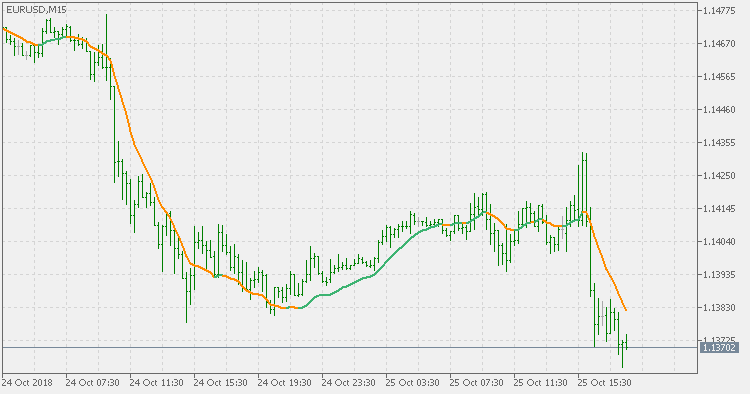Interesting script?
So post a link to it -
let others appraise it
You liked the script? Try it in the MetaTrader 5 terminal# Generalized DEMA - indicator for MetaTrader 5

|
Views:
3782
Rating:
(7)
Published:
2018.10.25 17:49
Updated:
2019.01.29 15:39

Theory:

The double exponential moving average (DEMA), was developed by Patrick Mulloy in an attempt to reduce the amount of lag time found in traditional moving averages. It was first introduced in the February 1994 issue of the magazine Technical Analysis of Stocks & Commodities in Mulloy's article "Smoothing Data with Faster Moving Averages.". The way to calculate is the following:

The Double Exponential Moving Average calculations are based combinations of a single EMA and double EMA into a new EMA:

1. Calculate EMA

2. Calculate Smoothed EMA by applying EMA with the same period to the EMA calculated in the first step

3. Calculate DEMA

DEMA = (2 * EMA) - (Smoothed EMA)

This version:

Instead of using fixed multiplication factor in the final DEMA formula, the generalized version allows you to change it. By varying the "volume factor" form 0 to 1 you apply different multiplications and thus producing DEMA with different "speed" - the higher the volume factor is the "faster" the DEMA will be (but also the slope of it will be less smooth). The volume factor is limited in the calculation to 1 since any volume factor that is larger than 1 is increasing the overshooting to the extent that some volume factors usage makes the indicator unusable.

Usage:

You can use it as any regular average or you can use the color change of the indicator as a signal.Connors_RSI

Connors RSI indicatorSimple_Decycler

Simple Decycler indicatorAvg_MA

Average from MA indicatorGeneralized double DEMA

Generalized double DEMA